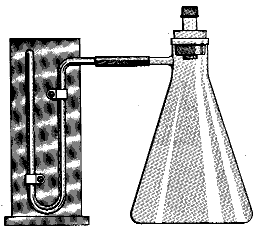# The Universal Gas Law Apparatus

$$\newcommand{\vecs}{\overset { \rightharpoonup} {\mathbf{#1}} }$$ $$\newcommand{\vecd}{\overset{-\!-\!\rightharpoonup}{\vphantom{a}\smash {#1}}}$$$$\newcommand{\id}{\mathrm{id}}$$ $$\newcommand{\Span}{\mathrm{span}}$$ $$\newcommand{\kernel}{\mathrm{null}\,}$$ $$\newcommand{\range}{\mathrm{range}\,}$$ $$\newcommand{\RealPart}{\mathrm{Re}}$$ $$\newcommand{\ImaginaryPart}{\mathrm{Im}}$$ $$\newcommand{\Argument}{\mathrm{Arg}}$$ $$\newcommand{\norm}{\| #1 \|}$$ $$\newcommand{\inner}{\langle #1, #2 \rangle}$$ $$\newcommand{\Span}{\mathrm{span}}$$ $$\newcommand{\id}{\mathrm{id}}$$ $$\newcommand{\Span}{\mathrm{span}}$$ $$\newcommand{\kernel}{\mathrm{null}\,}$$ $$\newcommand{\range}{\mathrm{range}\,}$$ $$\newcommand{\RealPart}{\mathrm{Re}}$$ $$\newcommand{\ImaginaryPart}{\mathrm{Im}}$$ $$\newcommand{\Argument}{\mathrm{Arg}}$$ $$\newcommand{\norm}{\| #1 \|}$$ $$\newcommand{\inner}{\langle #1, #2 \rangle}$$ $$\newcommand{\Span}{\mathrm{span}}$$$$\newcommand{\AA}{\unicode[.8,0]{x212B}}$$

Chemical Concepts Demonstrated

• The Dependence of P on n
• Dalton's Law of partial pressures
• Boyle's Law
• The dependence of P on T

## Demonstration

 Concept How it was demonstrated: Dependence of Pressure on Number of Moles 25 ml of H2 is injected into the apparatus. What happens to the the height of the column if an additional 25 ml is added? And another? Dalton's Law of Partial Pressures 25 mL of H2 is injected into the apparatus. What happens to the height of the column if 25mL of O2 is injected and mixed with the H2? Compare the mixture's height to the height of 50 mL of H2. Boyle's Law 25 mL of H2 gas is added to the 500mL and 2-L universal gas law apparatus. How do the different apparatus' compare? Dependence of Pressure on Temperature (Amonton's Law) At a constant volume and number of moles, the apparatus is heated. What happens to the height of the column as the temperature increases?

##Explanations

The pressure of a gas will increase as the number of moles of gas increases. The increase in the number of gas molecules within the container increases the frequency of collisions between the molecules and the walls of the container and will therefore increase the pressure.

The total pressure of a mixture of gases is the sum of the pressures of the individual components in the mixture. The pressure of the hydrogen and oxygen mixture will be equal to the sum of the pressure of the hydrogen gas alone plus the pressure of the oxygen gas alone.

Pressure increases as the volume of the container decreases. The 500 ml apparatus will have a higher pressure than the 2 L apparatus because of the difference in their volumes. A smaller volume allows the molecules to collide more frequently with each other and the walls of the container. Note that the product of the pressure times the volume of the apparatus is constant.

Pressure increases as temperature increases. This is due to the increase in the kinetic energy of the gas molecules within the apparatus. The faster the molecules are moving, the more force they exert on the wall of the container and again increase the pressure.

The Universal Gas Law Apparatus is shared under a CC BY-NC-SA 4.0 license and was authored, remixed, and/or curated by George Bodner.